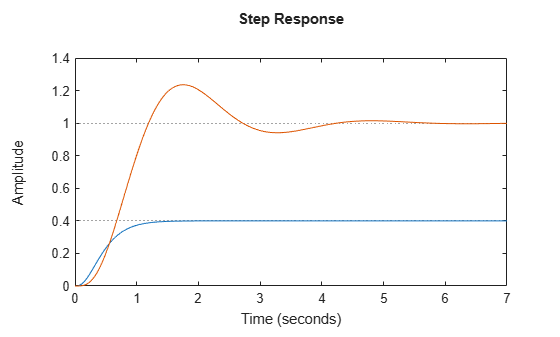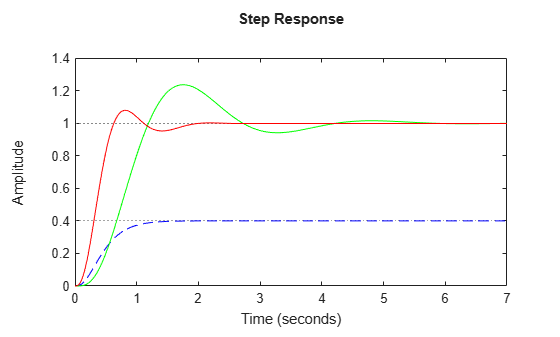## Time-Domain Responses of Multiple Models

This example shows how to compare the step responses of multiple models on a single plot using `step`. This example compares the step response of an uncontrolled plant to the closed-loop step response of the plant with two different PI controllers. You can use similar techniques with other response commands, such as `impulse` or `initial`, to obtain plots of responses of multiple models.

For this example, obtain two models whose time responses you want to compare, and plot them on a single step plot. For instance, you can compare a third-order plant `G`, and the closed-loop response of `G` with a controller `C1` having integral action.

```G = zpk([],[-5 -5 -10],100); C1 = pid(0,4.4); CL1 = feedback(G*C1,1); step(G,CL1);```When you provide multiple models to `step` as input arguments, the command displays the responses of both models on the same plot. If you do not specify a time range to plot, `step` attempts to choose a time range that illustrates the dynamics of all the models.

Compare the step response of the closed-loop model with another controller. Specify plot colors and styles for each response.

```C2 = pid(2.9,7.1); CL2 = feedback(G*C2,1); step(G,'b--',CL1,'g-',CL2,'r-')```You can specify custom plot color and style for each response in the plot. For example, `'g-'` specifies a solid green line for response `CL2`. For additional plot customization options, use `stepplot`.